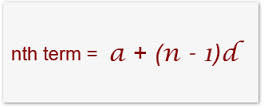# Sum of First 24 Terms

Algebra Level 2Find the sum of first 24 terms of the A.P. $a_1, a_2, a_3, a_4, \ldots$ if it is known that $a_1 + a_5 + a_{10} + a_{15} + a_{20} + a_{24}$ = 225.

×